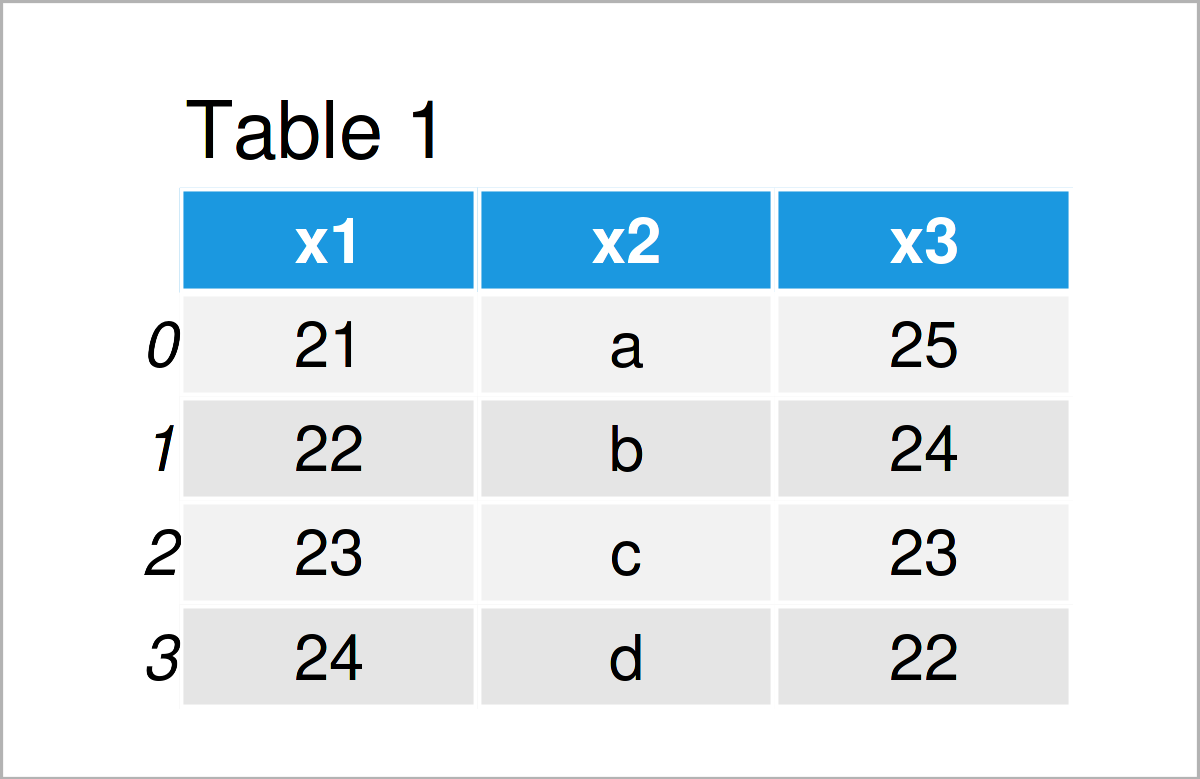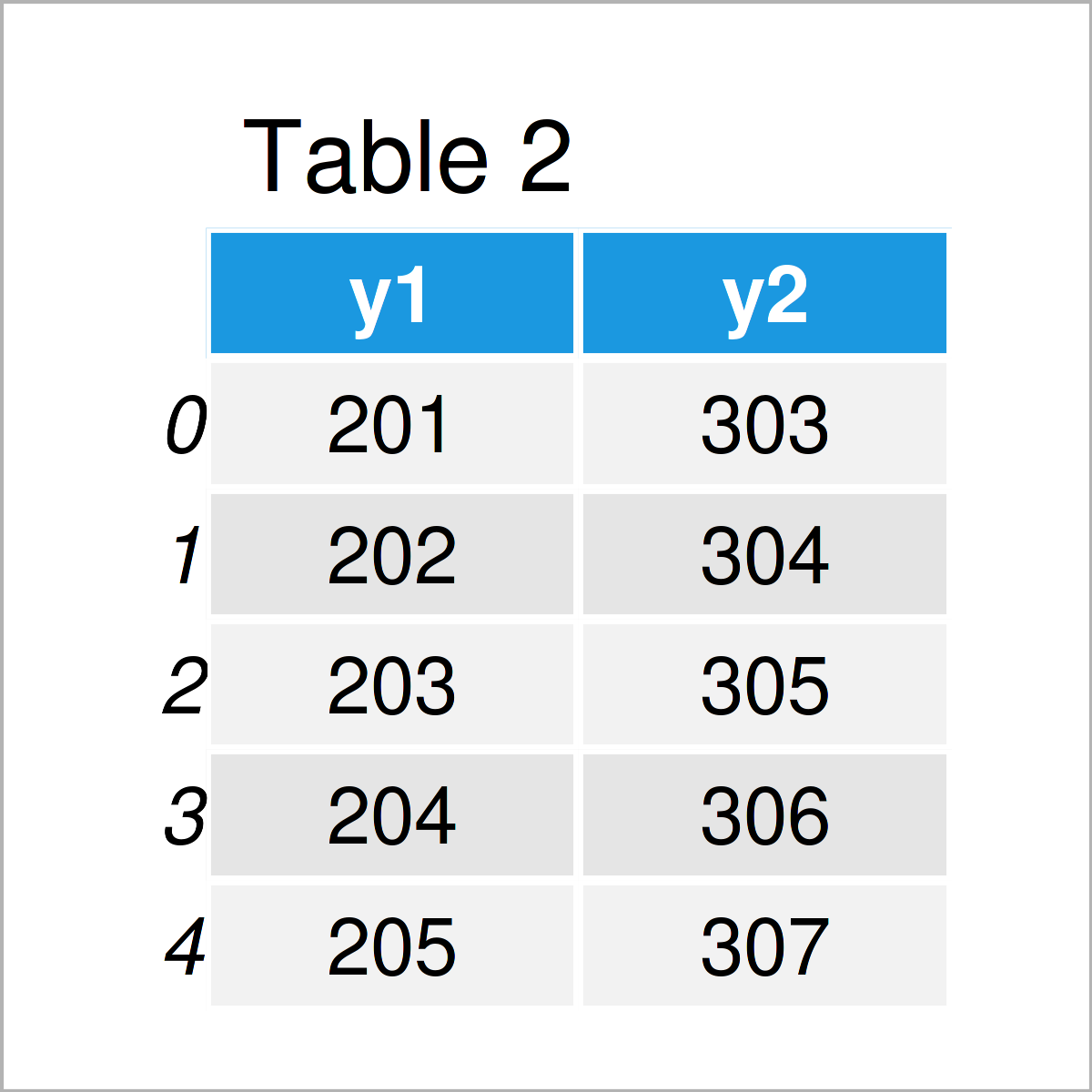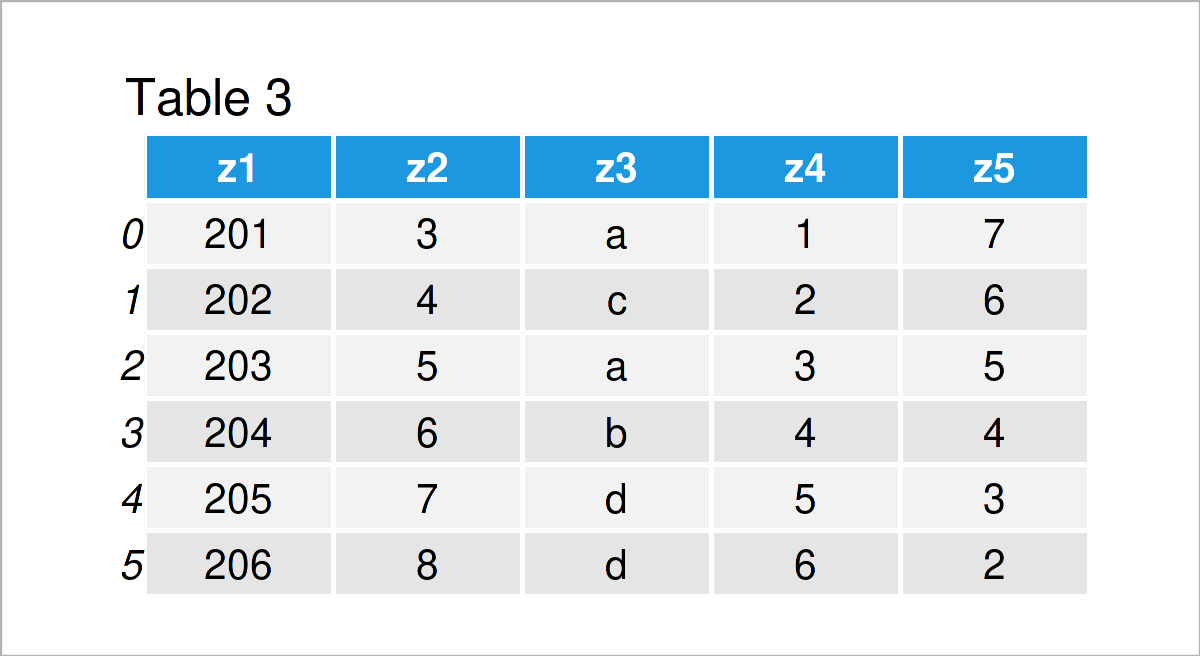# Write Multiple CSV Files in Python (Example)

On this page you’ll learn how to export several pandas DataFrames to multiple CSV files in the Python programming language.

Let’s dive right in!

## Example Data & Software Libraries

First, we need to load the pandas library:

`import pandas as pd                            # Import pandas`

We’ll use the following three pandas DataFrames as a basis for this tutorial:

```data1 = pd.DataFrame({'x1':range(21, 25),      # Create first pandas DataFrame
'x2':['a', 'b', 'c', 'd'],
'x3':range(25, 21, - 1)})
print(data1)                                   # Print first pandas DataFrame``````data2 = pd.DataFrame({'y1':range(201, 206),    # Create second pandas DataFrame
'y2':range(303, 308)})
print(data2)                                   # Print second pandas DataFrame``````data3 = pd.DataFrame({'z1':range(201, 207),    # Create third pandas DataFrame
'z2':range(3, 9),
'z3':['a', 'c', 'a', 'b', 'd', 'd'],
'z4':range(1, 7),
'z5':range(7, 1, - 1)})
print(data3)                                   # Print third pandas DataFrame```Have a look at the previous tables. They show our three exemplifying pandas DataFrames. Each of them consists of different values, rows, and columns.

## Example: Export pandas DataFrames to Multiple CSV Files Using for Loop

This example demonstrates how to save multiple pandas DataFrames to separate CSV files in Python.

For this task, we first have to create a list object that contains all the data sets that we want to write to a CSV output file:

```data_all = {                                   # Create list of all DataFrames
'data1': data1,
'data2': data2,
'data3': data3
}
print(data_all)                                # Print list of all DataFrames
# {'data1':    x1 x2  x3
# 0  21  a  25
# 1  22  b  24
# 2  23  c  23
# 3  24  d  22, 'data2':     y1   y2
# 0  201  303
# 1  202  304
# 2  203  305
# 3  204  306
# 4  205  307, 'data3':     z1  z2 z3  z4  z5
# 0  201   3  a   1   7
# 1  202   4  c   2   6
# 2  203   5  a   3   5
# 3  204   6  b   4   4
# 4  205   7  d   5   3
# 5  206   8  d   6   2}```

The previous output shows the printed list in the Python console. As you can see, we have created a list containing all of our three pandas DataFrames.

In the next step, we can use a for loop to print all our pandas DataFrames to multiple CSV files:

```for i in range(1, len(data_all) + 1):          # Write each DataFrame to separate CSV
data_i = data_all['data' + str(i)]
data_i.to_csv('data' + str(i) + '.csv')```

After executing the previous Python for loop, three new CSV files are appearing in our current working directory. The screenshot below illustrates how this working directory would look like:As you can see, the previously shown folder contains three different CSV files. Looks good!

## Video & Further Resources

I have recently published a video on my YouTube channel, which illustrates the Python programming codes of this tutorial. You can find the video below:

In addition, you may want to read the related tutorials on my homepage.

In this tutorial, I have illustrated how to save and download different pandas DataFrames to multiple CSV files in the Python programming language. Let me know in the comments section below, if you have further questions.

Subscribe to the Statistics Globe Newsletter